##全国校区# 手把手教你用R语言读取CSV文件2020-08-24http://www.jaredlander.com/data/TomatoFirst.csv

```> theUrl <-"http://www.jaredlander.com/data/TomatoFirst.csv"
```

`> head(tomato)`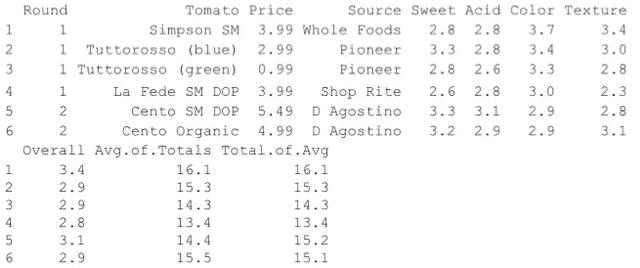stringAsFactors参数也可以用在data.frame中。再次创建“Sport”列。

```> x <- 10:1
> y <- -4:5
> q <- c("Hockey", "Football", "Baseball", "Curling", "Rugby",
+        "Lacrosse", "Basketball", "Tennis", "Cricket", "Soccer")
> theDF <-data.frame(First=x, Second=y, Sport=q, stringsAsFac=FALSE)
> theDF\$Sport
```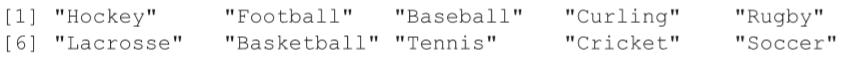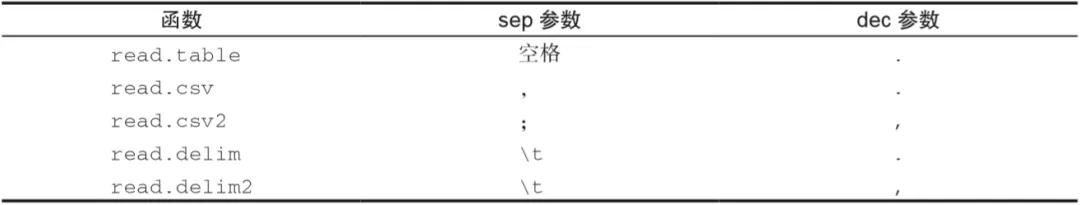▲表6-1 读取大文本文件的函数及其默认参数

```> library(readr)
> theUrl <- "http://www.jaredlander.com/data/TomatoFirst.csv"
Parsed with column specification:
cols(
Round = col_integer(),
Tomato = col_character(),
Price = col_double(),
Source = col_character(),
Sweet = col_double(),
Acid = col_double(),
Color = col_double(),
Texture = col_double(),
Overall = col_double(),
`Avg of Totals` = col_double(),
`Total of Avg` = col_double()
)```

`> tomato2`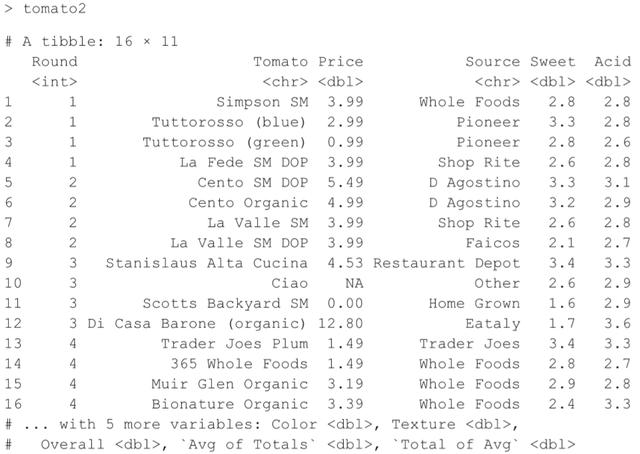```> library(data.table)
> theUrl <- "http://www.jaredlander.com/data/TomatoFirst.csv"
```

```> head(tomato3)
```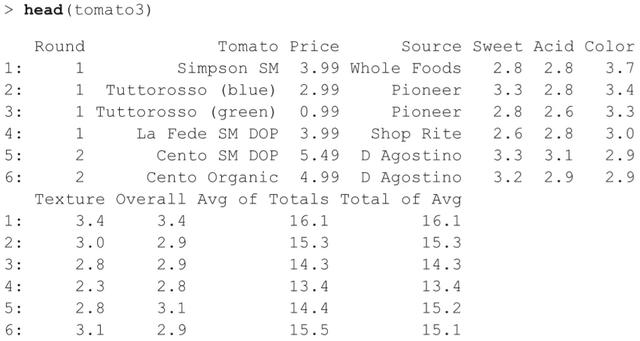OK